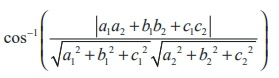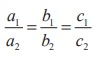Home | | Maths 12th Std | Angle between two planes

# Angle between two planes

The angle between two given planes is same as the angle between their normals.

Angle between two planes

The angle between two given planes is same as the angle between their normals.

### Proof

If θ is the acute angle between two planes1  = p1 and2  = p2  , then θ is the acute angle between their normal vectors1 and2

Therefore,Remark### Theorem 6.19

The acute angle θ between the planes a1x + b1y + c1z + d1 = 0 and a2b2c2d2 = 0 is given by### Proof

If1 and2 are the vectors normal to the two given planes a1x + b1y + c1z + d1 = 0 and a2x + b2y + c2z + d2 = 0 respectively. Then,Therefore, using equation (1) in theorem 6.18 the acute angle θ between the planes is given by### Remark

(i) The planes a1x + b1y + c1z + d1 = 0 and a2x + b2y + c2z + d2 = 0 are perpendicular if a1a2  + b1b2   + c1c2  = 0

(ii) The planes a1x + b1 y + c1z + d1 = 0 and a2x + b2y + c2z + d2 = 0 are parallel if(iii) Equation of a plane parallel to the plane ax + by + cz = p is ax + by + cz = k , k R.

### Example 6.47

Find the acute angle between the planes.(2 ˆi + 2ˆ j + 2ˆk ) = 11 and 4x - 2 y + 2z = 15

### Solution

The normal vectors of the two given planes= (2 ˆi + 2ˆ j + 2ˆk ) = 11 and 4x - 2 y + 2z = 15 are= 2ˆi + 2ˆ j + 2ˆk and= 4ˆi - 2ˆ j + 2ˆk  respectively.

If θ is the acute angle between the planes, then we haveTags : Definition, Theorem, Proof, Solved Example Problems, Solution , 12th Mathematics : UNIT 6 : Applications of Vector Algebra
Study Material, Lecturing Notes, Assignment, Reference, Wiki description explanation, brief detail
12th Mathematics : UNIT 6 : Applications of Vector Algebra : Angle between two planes | Definition, Theorem, Proof, Solved Example Problems, Solution